# Symplectic cohomology

Flöer cohomology of symplectic manifolds

While standard cohomology is very useful to answer questions about the zeros of vector fields, fixed points of diffeomorphisms, and the points of intersection of a pair of submanifolds of complementary dimension, symplectic cohomology is supposed to refine such answers in the case of symplectic manifolds, Hamiltonian vector fields, symplectic diffeomorphisms and Lagrangean submanifolds.

Symplectic cohomology came out from the work of A. Flöer [a3], [a4] on the Arnol'd conjecture (concerning the minimal number of fixed points of a symplectic diffeomorphism), which can be reformulated as one of the above questions, and is mostly known in the literature as Flöer cohomology of symplectic manifolds.

One can define symplectic cohomology for a symplectic manifold $( M , \omega )$, for a symplectic diffeomorphism $\phi : ( M , \omega ) \rightarrow ( M , \omega )$ and for a pair of transversal Lagrangeans $( L _ { + } , L _ { - } )$ in $( M , \omega )$. Below, the first is denoted by $\operatorname{SH} ^ { * } ( M , \omega )$, the second by $\operatorname{SH} ^ { * } ( M , \omega , \phi )$ and the third by $\operatorname {SH} ^ { * } ( M , \omega , L _ { + } , L _ { - } )$. The Euler characteristic of $\operatorname{SH} ^ { * } ( M , \omega )$, $\operatorname{SH} ^ { * } ( M , \omega , \phi )$, and $\operatorname {SH} ^ { * } ( M , \omega , L _ { + } , L _ { - } )$ are the standard Euler characteristic of $M$, the Lefschetz number of $\phi$ and the standard intersection number of $L _ { + }$ and $L_{-}$ in $M$, respectively (cf. Intersection theory). When $\phi$ is symplectically isotopic to $\operatorname{id}$, $\operatorname{SH} ^ { * } ( M , \omega ) = \operatorname{SH} ^ { * } ( M , \omega , \phi )$ and when $( N , \tilde{\omega} ) = ( M , \omega ) \times ( M , - \omega )$, $L _ { + }$ is the diagonal in $M \times M$ and $L_{-}$ is the graph of $\phi$, $\operatorname{SH} ^ { * } ( M , \omega , \phi ) = \operatorname{SH} ^ { * } ( N , \tilde { \omega } , L _ { + } , L - )$.

One can define pairings

\begin{equation*} \operatorname{SH} ^ { * } ( M , \omega , L _ { 1 } , L _ { 2 } ) \bigotimes \operatorname{SH} ^ { * } ( M , \omega , L _ { 2 } , L _ { 3 } ) \rightarrow \operatorname{SH} ^ { * } ( M , \omega , L _ { 1 } , L _ { 3 } ), \end{equation*}

\begin{equation*} \operatorname{SH} ^ { * } ( M , \omega , \phi _ { 1 } ) \bigotimes \operatorname{SH} ^ { * } ( M , \omega , \phi _ { 2 } ) \rightarrow \operatorname{SH} ^ { * } ( M , \omega , \phi _ { 2 } . \phi _ { 1 } ) \end{equation*}

and

\begin{equation*} \operatorname{SH} ^ { * } ( M , \omega ) \bigotimes \operatorname{SH} ^ { * } ( M , \omega ) \rightarrow \operatorname{SH} ^ { * } ( M , \omega ). \end{equation*}

The last pairing provides an associative product, known as the pair of pants product, hence a ring structure on $\operatorname{SH} ^ { * } ( M , \omega )$, cf. the section "Pair of pants product" below.

As a group, $\operatorname{SH} ^ { * } ( M , \omega )$ is isomorphic to $H ^ { * } ( M ; \mathbf{Z} )$ and when $\phi$ is symplectically isotopic to $\operatorname{id}$, $SH ^ { * } ( M , \omega , L , \phi ( L ) )$ is isomorphic to $H ^ { * } ( L ; \mathbf{Z} )$, properly regraded. In the first case the "pair of pants" product is different from the cup product (cf. Cohomology) and the deviation of one from the other is measured by numerical invariants associated to the symplectic manifold $( M , \omega )$, the so-called Gromov–Witten invariants (cf. [a1], Chap. 7).

With this ring structure the symplectic cohomology $\operatorname{SH} ^ { * } ( M , \omega )$ identifies to the quantum cohomology ring of the symplectic manifold (cf. [a1], Chap. 10).

The symplectic cohomologies mentioned above are not defined for all symplectic manifolds for technical reasons (cf. Definition c) below). The largest class of symplectic manifolds for which $\operatorname{SH} ^ { * } ( M , \omega )$ is defined in [a1] is the class of weakly symplectic manifolds. Recently, (cf. [a6]) it was extended to all symplectic manifolds.

## Definitions.

The definitions below are essentially due to Flöer (cf. [a3], [a4]) in the case $( M , \omega )$ is monotonic and ameliorated and have been extended by others. This presentation closely follows [a1].

For a symplectic manifold $( M , \omega )$ one says that the almost-complex structure (i.e., an automorphism of the tangent bundle whose square is $- \operatorname{ Id }$) $J$ tames $\omega$ if $\omega ( J u , J v ) = \omega ( u , v )$, $\omega ( v , J v ) > 0$ for any $u , v \in T M$. Such a $J$ defines a Riemannian metric $g ( \omega , J )$. The space of all almost-complex structures which tame $\omega$ is contractible (cf. also Contractible space), therefore the $J$s provide isomorphic complex vector bundle structures on $T M$. Denote by $c _ { 1 } \in H ^ { 2 } ( M ; \mathbf{Z} )$ the first Chern class of $T M$.

An element $A \in H _ { 2 } ( M ; \mathbf{Z} )$ is called spherical if it lies in the image of the Hurewicz isomorphism (cf. Homotopy group). Denote by $N$ the smallest absolute value $c_ { 1 } ( A )$.

The symplectic manifold is called monotonic if there exists a $\lambda > 0$ so that $\omega ( A ) = \lambda c _ { 1 } ( A )$ for $A$ spherical, and weakly monotonic if it is either monotonic, or $c _ { 1 } ( A ) = 0$ for $A$ spherical, or $N \geq n - 2$, with $n = \operatorname { dim } M / 2$.

Given a symplectic manifold $( M , \omega )$, choose an almost-complex structure $J$ which tames $\omega$ and a ($1$-periodic) time-dependent potential $H : S ^ { 1 } \times M \rightarrow \mathbf{R}$, $S ^ { 1 } = \mathbf{R} / \mathbf{Z}$. Let $c _ { 1 } \in H ^ { 2 } ( M ; \mathbf{Z} )$ be the first Chern class of $( T M , J )$ and denote by $P$ the space of ($1$-periodic) closed curves $x : S ^ { 1 } \rightarrow M$ which are homotopically trivial. Consider the covering $\tilde{P}$ of $P$ whose points are equivalence classes of pairs $\tilde{x} = ( x , u )$, $u : D ^ { 2 } \rightarrow M$, $u | _ { \partial D ^ { 2 } } = x$, with the equivalence relation $( x , u ) \equiv ( x ^ { \prime } , u ^ { \prime } )$ if and only if $x = x ^ { \prime }$ and. Here,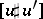represents the $2$-cycle obtained by putting together the $2$-chains $u ( D ^ { 2 } )$ and $- u ^ { \prime } ( D ^ { 2 } )$. Define $S _ { H } : \tilde{P} \rightarrow \mathbf{R}$ by the formula

\begin{equation} \tag{a1} S _ { H } ( \tilde{x} ) = \int _ { D ^ { 2 } } u ^ { * } ( \omega ) + \int _ { 0 } ^ { 1 } H ( t , x ( t ) ) d t, \end{equation}

and observe that the critical points of $S _ { H }$ are exactly all $\tilde{x} = ( x , u )$ with $x$ a $1$-periodic trajectory of the Hamiltonian system associated to $H$. Denote the set of such critical points by $X$. When $H$ is generic, all critical points are non-degenerate but of infinite Morse index. Fortunately, there exists a $\alpha _ { H } : X \rightarrow \mathbf{Z}$, the Connely–Zehnder version of the Maslov index, cf. [a2], so that for any two critical points $\tilde{x}$, $\tilde{y}$, the difference $\alpha _ { H } ( \tilde{y} ) - \alpha _ { H } ( \tilde { x } )$ behaves as the difference of Morse indices in classical Morse theory. More precisely, one can prove that:

a)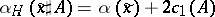for any spherical class $A \in H _ { 2 } ( M ; \mathbf{Z} )$. Here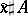denotes the class represented by $( x , u \sharp v )$ with $v : S ^ { 2 } \rightarrow M$ representing $A$.

b) If $\tilde{x}_ - = ( x_ - , u_ - )$ and $\tilde { x }_{ + }= ( x _ { + } , u _{+} )$ are two critical points, then the mappings $w : \mathbf{R} \times S ^ { 1 } \rightarrow M$ with the property that $\operatorname { lim } _ { s \rightarrow \pm \infty } w ( s , t ) = x _ { \pm } ( t )$ and $( x _ { + } , u _ { - } \sharp w ) \equiv \tilde{x} _ { + }$, which satisfy the perturbed Cauchy–Riemann equations

\begin{equation} \tag{a2} \frac { \partial w } { \partial s } + J ( u ) \frac { \partial w } { \partial t } = \nabla H ( t , w ( s , t ) ), \end{equation}

$s \in \mathbf{T}$, $t \in S ^ { 1 }$, are trajectories for $\operatorname { grad } S _ { H }$ from $\tilde{x}_-$ to $\tilde { x } _ { + }$, where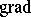is taken with respect to the $L_{2}$ metric induced from the Riemannian metric $g ( \omega , J )$ on $M$. cf. [a4]. Here, $u_{ -} \sharp$ is the obvious extension of $x_{+}$ provided by $u_-$ and $w$ and $\nabla$ denotes the gradient on $M$ with respect to $g ( \omega , J )$. For generic $J$ and $H$, the space of these mappings, denoted by $\mathcal{M} ( \tilde { x } _ { - } , \tilde { x } _ { + } )$, is a smooth manifold of dimension $\alpha _ { H } ( \tilde{x} _ { + } ) - \alpha _ { H } ( \tilde{x} _ { - } )$ with $\mathbf{R}$ acting freely on it (by translations on the parameter $s$).

c) For $( M , \omega )$ weakly monotonic, $\alpha _ { H } ( \tilde { x } _ { + } ) - \alpha _ { H } ( \tilde { x } _ { - } ) = 1$, ${\cal M} ( \tilde { x } _ { + } , \tilde { x } _ { - } ) / \bf R$ is compact; hence, when $J$ and $H$ generic, finite. Even more, in this case for any real number $c$,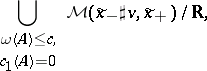with $A$ a spherical class in $H _ { 2 } ( M ; \mathbf{Z} )$, is compact, hence finite. The proof of c) relies on Gromov's theory of pseudo-holomorphic curves in symplectic manifolds, cf. [a5] or [a1] for more details.

As in Morse theory one can construct a cochain (or chain) complex generated by the points in $X$, graded with the help of $\alpha _ { H }$ and with coboundary given by the "algebraic" cardinality of the finite set $\mathcal{M} ( \tilde { x } , \tilde { y } ) / \mathbf{R}$, when $\alpha _ { H } ( \tilde{y} ) - \alpha _ { H } ( \tilde{x} ) = 1$. Actually, c) permits one to "complete" this complex to a cochain complex of modules over the Novikov ring associated to $( M , \omega )$ (cf. [a1], Chap. 9). The cohomology of this complex is independent of $J$ and $H$ and is the symplectic cohomology.

In the case of Lagrangean submanifolds $L_{-}$ and $L _ { + }$, the space $P$ consists of paths $x: [ 0,1 ] \rightarrow M$ with $x ( 0 ) \in L _ { - }$ and $x ( 1 ) \in L _ { + }$. There is no Hamiltonian, the functional $S$ is the symplectic action and (a2) become the Cauchy–Riemann equations. A function like $\alpha _ { H }$ is not naturally defined, but the difference index of two critical points, an analogue of $\alpha _ { H } ( \tilde{y} ) - \alpha _ { H } ( \tilde { x } )$ can be defined and is given by the classical Maslov index (cf. Fourier integral operator). There is no natural $\bf Z$-grading in this case but there is a natural $2 N$-grading with $N$ as defined at the beginning.

## Pair of pants product.

Consider a Riemann surface $\Sigma$ of genus zero with $3$ punctures. Choose a conformal parametrization of each of its three ends, $\varphi _ { 1 } , \varphi _ { 2 } : ( - \infty , 0 ) \times S ^ { 1 } \rightarrow \Sigma$ and $\varphi _ { 3 } : ( \infty , 0 ) \times S ^ { 1 } \rightarrow \Sigma$, with $U _ { i } = \varphi _ { i } ( ( \pm \infty , 0 ) \times S ^ { 1 } )$ disjoint, and put $\widetilde { \Sigma } = \Sigma \backslash \cup _ { i = 1,2,3 } U _ { i }$. Choose an almost-complex structure $J$ on $M$ which tames $\omega$ and a smooth mapping $H : \Sigma \times M \rightarrow \mathbf{R}$ with $H$ restricted to $U_i$ constant in $s$ ($s \in ( \pm \infty , \pm 1 )$), and $H$ restricted to $\widetilde{ \Sigma }$ being zero. Put $H _ { i } ( t , m ) = H ( \varphi _ { i } ( s , t ) , m )$, $t \in S ^ { 1 }$, $m \in M$.

Let $\tilde { x } _ { i } = ( x _ { i } , u _ { i } )$ be a critical point of $S _ { H _ { i } }$. Consider all mappings $\sigma : \Sigma \rightarrow M$ with $\operatorname { lim } _ { s \rightarrow \pm \infty } ( \sigma \cdot \varphi _ { i } ( s , t ) ) = x _ { i } ( t )$, $i = 1,2,3$, and $( x _ { 3 } , u _ { 1 } \cup u _ { 2 } \cup \sigma ) \equiv ( x _ { 3 } , u _ { 3 } )$, which restricted to $U_i$ satisfy the perturbed Cauchy–Riemann equations (a2) for $H _ { i }$ and when restricted to $\widetilde{ \Sigma }$ are pseudo-holomorphic curves. Here, $u _ { 1 } \cup u _ { 2 } \cup \sigma : D ^ { 2 } \rightarrow M$ denotes the mapping obtained from $u_1$, $u_2$ and $w$ in the obvious way.

The theory of pseudo-holomorphic curves implies that when $( M , \omega )$ is weakly monotonic and $H _ { i }$, $i = 1,2$, $J$ are generic, the space of these mappings is a smooth manifold of dimension $\alpha _ { H _ { 3 } } - \alpha _ { H _ { 2 } } - \alpha _ { H _ { 1 } }$, while if this dimension is zero, this space is compact hence finite. Using the "algebraic" cardinality of these sets, one can define a pairing of the cochain complexes associated to $( H _ { 1 } , J )$ and $( H _ { 1 } , J )$ into the cochain complex associated to $( H _ { 3 } , J )$ (cf. [a1], Chap. 10). Since the cohomology of these complexes is independent of $H _ { i }$, this pairing induces a pairing of $\operatorname{SH} ^ { * } ( M , \omega )$ and $\operatorname{SH} ^ { * } ( M , \omega )$ into $\operatorname{SH} ^ { * } ( M , \omega )$, which turns out to be an associative product and is called the pair of pants product.

How to Cite This Entry:
Symplectic cohomology. Encyclopedia of Mathematics. URL: http://encyclopediaofmath.org/index.php?title=Symplectic_cohomology&oldid=50589
This article was adapted from an original article by D. Burghelea (originator), which appeared in Encyclopedia of Mathematics - ISBN 1402006098. See original article